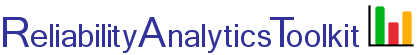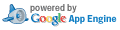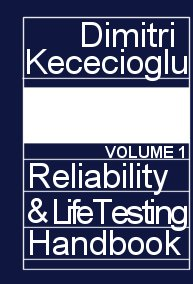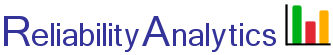# Reliability Growth Planning

This tool is intended to assist in developing an idealized reliability growth curve using methods described in MIL-HDBK-189. The input table below is pre-filled with an example test data set, "example of case 2," found on page 46 of from MIL-HDBK-189 (Ref. 1). For a given initial test period (phase 1) and associated average MTBF and growth rate, the tool calculates an idealized reliability growth curve that can be expected from subsequent test phases.

By selecting plot option two below, the tool calculates the test time required to achieve an MTBF goal based on the phase 1 parameters entered in the first line of the table. For example, the pre-populated test data set results in an achieved MTBF of 110 hours after 10,000 hours of testing. Entering only the phase 1 information from the test data set and selecting plot option 2 with an MTBF goal of 110 hours, results in 10,000 hours of test time required (subject to rounding errors), as expected.

## Calculation Inputs:

Test Phase
(i)
Hours Per Test Phase
Expected In-Phase Growth Rate (α)MTBF at Start of Test Phase i
1
2 same as phase 1calculated
3 same as phase 1calculated
4 same as phase 1calculated
5 same as phase 1calculated
6 same as phase 1calculated
7 same as phase 1calculated
8 same as phase 1calculated
9 same as phase 1calculated

Ref. 2, pp 32, quotes historical growth rates in the range of 0.23 to 0.53.
Ref. 4, pp 82 , quotes growth rates in the range of 0.05 for in-service improvements to 0.41 for dedicated development testing.

Decimal places:

Plot options:

1. Plot showing MTBF after each phase defined above.
2. Using the data entered for Test Phase 1 above, plot the test time needed to reach an MTBF goal of hours.

## Featured Reference:Reliability and Life Testing Handbook, Vol. 1

Toolkit Home